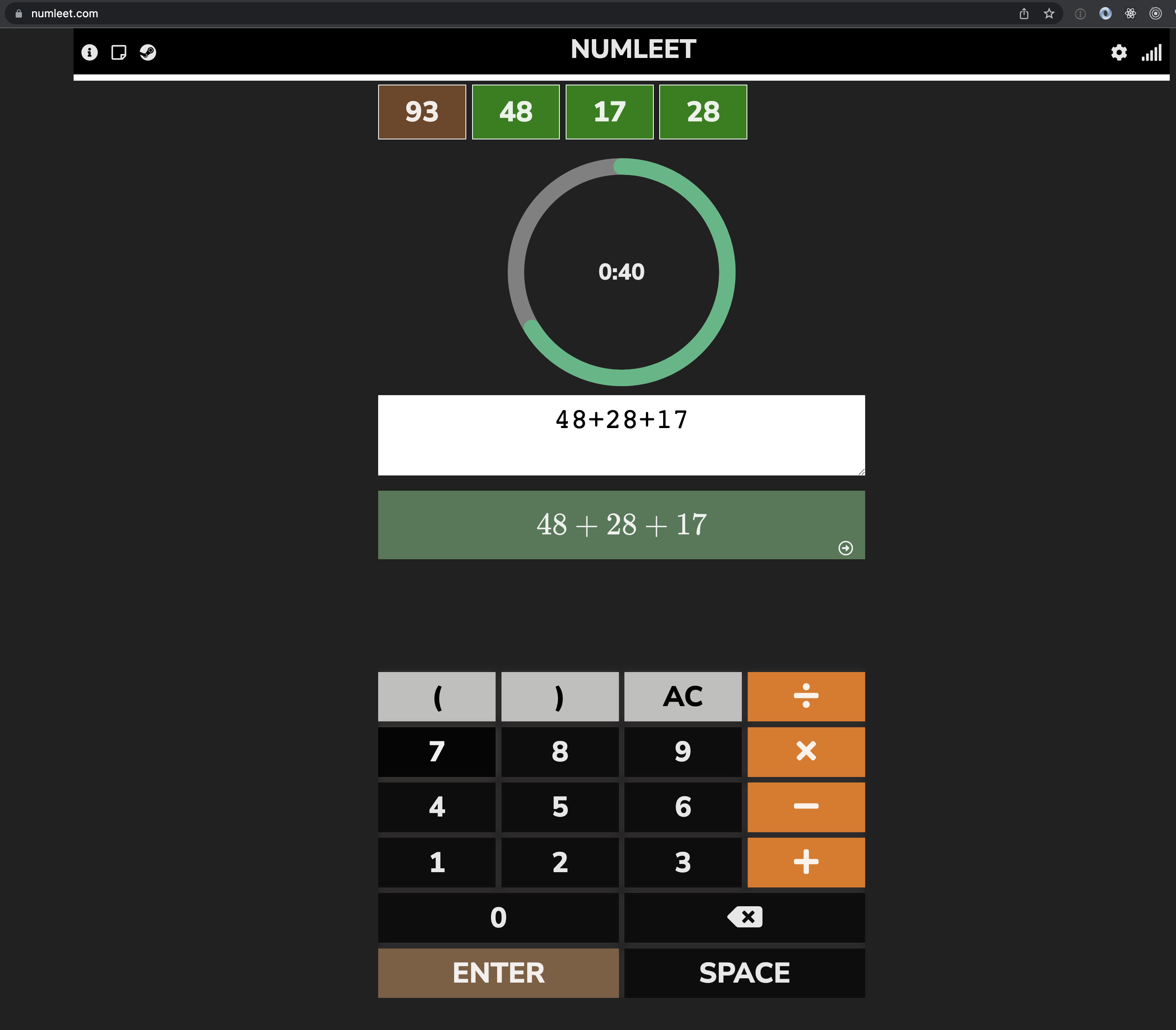# Numleet

Math game that challenges you to find correct equation in 1m
0 reviews
Do you use Numleet?
What is Numleet?
Numleet is a math thinking game where you need to derive the equations from the answer and numbers supplied. For instance, you can get (easy example) 10, 6, 2, 2 and two correct solutions would be 6 * 2 - 2 or 6 + 2 + 2. Levels choices are included

### Recent launches

Numleet
Numleet is a math thinking game where you need to derive the equations from the answer and numbers supplied. For instance, you can get (easy example) 10, 6, 2, 2 and two correct solutions would be 6 * 2 - 2 or 6 + 2 + 2. Levels choices are included